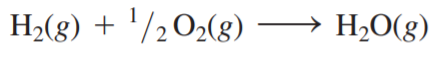×
Get Full Access to Chemistry: A Molecular Approach - 3 Edition - Chapter 9 - Problem 105e
Get Full Access to Chemistry: A Molecular Approach - 3 Edition - Chapter 9 - Problem 105e

×

# If hydrogen were used as a fuel, it could be burnedISBN: 9780321809247 1

## Solution for problem 105E Chapter 9

Chemistry: A Molecular Approach | 3rd Edition

• Textbook Solutions
• 2901 Step-by-step solutions solved by professors and subject experts
• Get 24/7 help from StudySoup virtual teaching assistantsChemistry: A Molecular Approach | 3rd Edition

4 5 1 258 Reviews
13
3
Problem 105E

If hydrogen were used as a fuel, it could be burned according to this reaction:Use average bond energies to calculaterxn for this reaction and also for the combustion of methane (CH4). Which fuel yields more energy per mole? Per gram?

Step-by-Step Solution:

Solution 105E Step 1 of 4 For the reaction Thus we can see that at the end of this reaction one HH bond and 1/2 O=O bond are broken and 2 bonds OH are formed.We have to remember that when a reaction happens, we have to spend energy to break bonds (positive H ), while broken bonds release energy (negative H) Since For H-H H = 436 kJ/mol For O=O H = 498 kJ/mol Thus H broken bonds= (H H-H)+ 1/2 (H O=O)= 685 kJ/mol For O-H H = -464 kJ/mol Thus H formed bonds= 2(H O-H)= -928 kJ/mol Since H RXN (H broken bonds) + (H formed bonds) H RXN (685 kJ/mol) + (- 928 kJ/mol)= -243 kJ/mol This means that 1 mol of H re2ases 243 kJ.

Step 2 of 4

Step 3 of 4

##### ISBN: 9780321809247

This full solution covers the following key subjects: Fuel, reaction, according, Bond, burned. This expansive textbook survival guide covers 82 chapters, and 9454 solutions. The full step-by-step solution to problem: 105E from chapter: 9 was answered by , our top Chemistry solution expert on 02/22/17, 04:35PM. Since the solution to 105E from 9 chapter was answered, more than 898 students have viewed the full step-by-step answer. Chemistry: A Molecular Approach was written by and is associated to the ISBN: 9780321809247. This textbook survival guide was created for the textbook: Chemistry: A Molecular Approach, edition: 3. The answer to “?If hydrogen were used as a fuel, it could be burned according to this reaction: Use average bond energies to calculate rxn for this reaction and also for the combustion of methane (CH4). Which fuel yields more energy per mole? Per gram?” is broken down into a number of easy to follow steps, and 42 words.

Unlock Textbook Solution